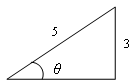# Sine function

To use this function, choose Calc > Calculator.

The sine of an acute angle of a right triangle is the ratio of the opposite leg to the hypotenuse (the longest side, opposite the right angle), measured in radians.The sine of angle θ is the ratio 3/5, or 0.6.

The sine of θ can also be defined as the y-coordinate of the point on the unit circle that is located at an angle θ from the origin (measured counterclockwise from the positive x-axis).